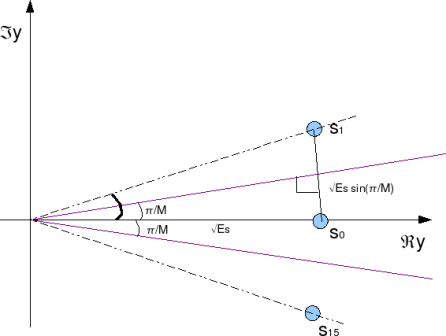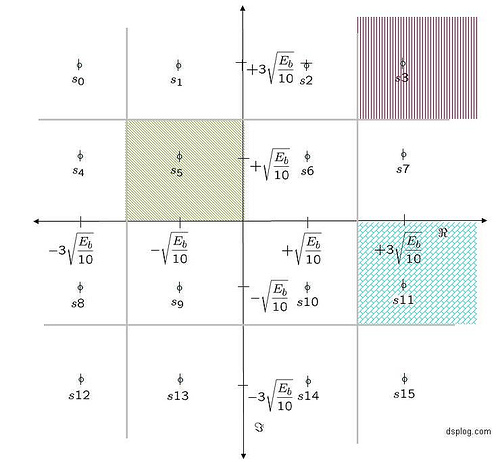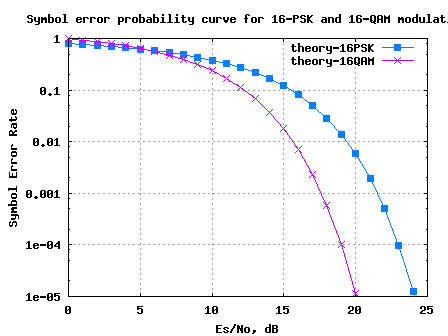- DSP log - http://www.dsplog.com -

Comparing 16PSK vs 16QAM for symbol error rate

Posted By Krishna Sankar On March 29, 2008 @ 6:05 am In Modulation | 56 Comments

In two previous posts, we have derived theoretical symbol error rate for 16-QAM and 16-PSK modulation schemes. The links are:

Given that we are transmitting the same number of constellation points in both 16-PSK and 16-QAM, let us try to understand the better modulation scheme among the two, i.e. to answer the following question:

For the same signal to noise ratio , will 16-PSK or 16-QAM give a lower symbol error rate?

## Distance between the constellation points for 16-PSKFigure: Distance between constellation points for 16-PSK modulation

As can be seen from the above figure, the distance between symbols and can be approximated as,
, where .

## Distance between the constellation points for 16-QAMFigure: Distance between constellation points for 16-QAM modulation

As can be seen from the above figure, the distance between the constellation points for 16QAM modulation is,

.

Comparing both,
.

The distance between the constellation points fo 16QAM modulation is around 1.6x the value for 16PSK modulation. Expressing in dB’s, this comes to around .

More the distance between the constellation, lesser is the chance of a constellation point getting decoded incorrectly. This implies that for the same symbol error rate, 16QAM modulation requires only 4.19dB lesser signal to noise ratio , when compared with 16PSK modulation.

 % Matlab/Octave code for comparing the symbol error rate for 16PSK and 16QAM modulation clear M = 16; Es_N0_dB = [0:25]; % multiple Es/N0 values theorySer_16PSK = erfc(sqrt(10.^(Es_N0_dB/10))*sin(pi/M)); theorySer_16QAM = 3/2*erfc(sqrt(0.1*(10.^(Es_N0_dB/10)))); close all figure semilogy(Es_N0_dB,theorySer_16PSK,'bs-','LineWidth',2); hold on semilogy(Es_N0_dB,theorySer_16QAM,'mx-','LineWidth',2); axis([0 25 10^-5 1]) grid on legend('theory-16PSK', 'theory-16QAM'); xlabel('Es/No, dB') ylabel('Symbol Error Rate') title('Symbol error probability curve for 16-PSK and 16-QAM modulation')Figure: Symbol Error Rate for 16PSK and 16QAM modulation

As can be observed, at a symbol error rate of , 16QAM requires only arond 19dB whereas 16PSK requires around 23dB of .

No wonder we find 16-QAM modulation instead of 16-PSK in typical specifications like IEEE-802.11a , IEEE802.16d  etc.Hope this helps.

Thanks,

Krishna

URL to article: http://www.dsplog.com/2008/03/29/comparing-16psk-vs-16qam-for-symbol-error-rate/

URLs in this post:

 Symbol error rate for 16-PSK: http://www.dsplog.com/2008/03/18/symbol-error-rate-for-16psk/

 Symbol error rate for 16-QAM: http://www.dsplog.com/2007/12/09/symbol-error-rate-for-16-qam/